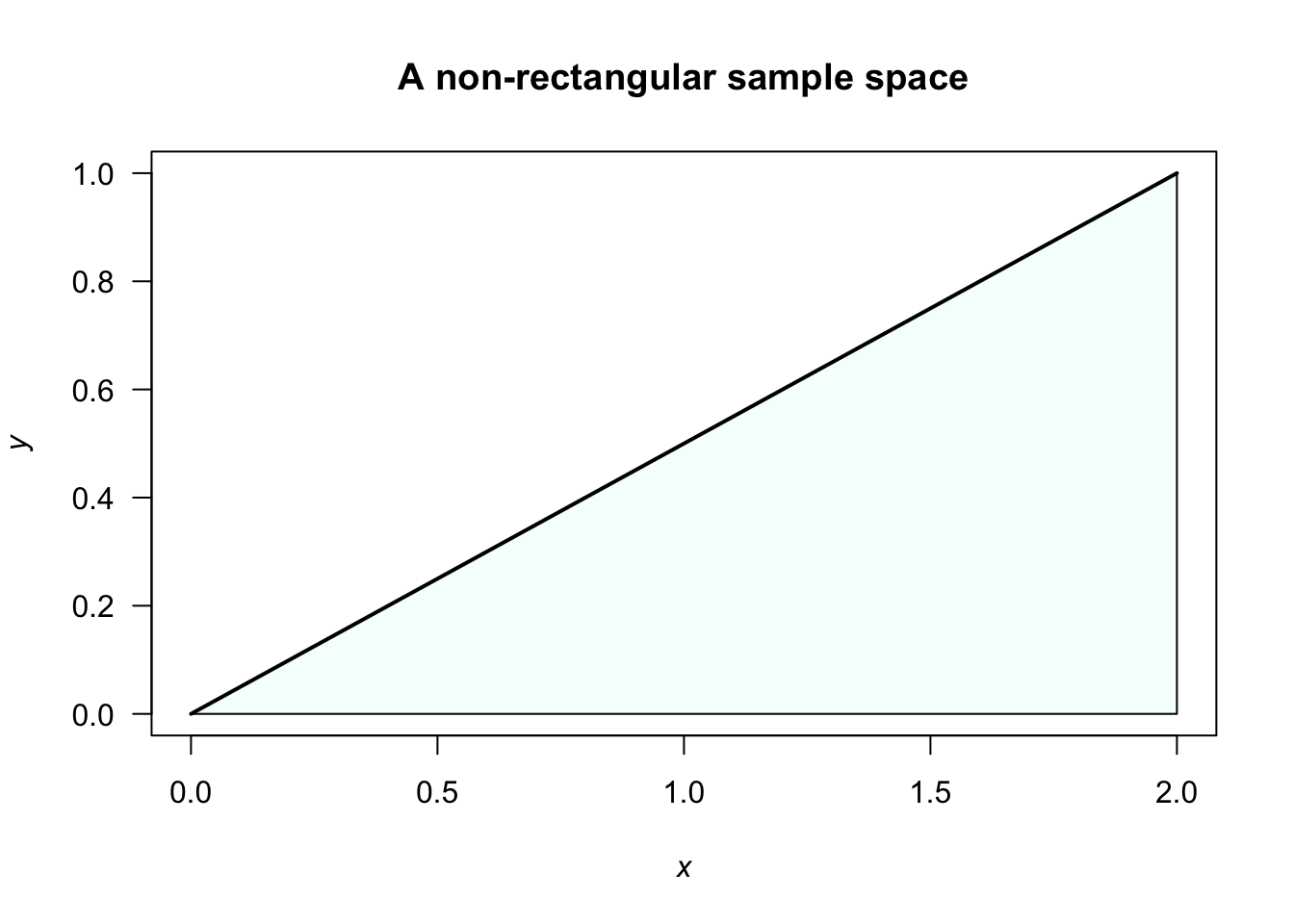## 2.4 Marginal distributions

A marginal distribution can be found by ‘integrating out’ (in the continuous case) the other variables. You can imagine looking at the joint probability function in (say) the $$x$$-direction, and accumulating the probability in that direction.

Example 2.8 (Rectangular sample space) For the joint probability distribution above, the marginal distribution for $$X$$ is $f_X(x) = \int_{-1}^1 \frac{3(x + z^2)}{5}\, dz = \frac{2(3x+1)}{5}$ for $$0<x<1$$. Note that this is a valid PDF.

Similarly, $f_Z(z) = \int_0^1 \frac{3(x + z^2)}{5}\, dx = \frac{3(1+2z^2)}{10}$ for $$-1<z<1$$.
Example 2.9 (Non-rectangular sample space) Consider the PDF for two rvs $$X$$ and $$Y$$: $f_{X,Y}(x, y) = k (x+2y)\qquad\text{for 0<x<2 and 0<y<(x/2)}.$ To find the value of $$k$$, we need to ensure the integral over the sample space $$S$$ is one. Note that the sample space here is non-rectangular, so we need to be careful with the limits of integration; see Fig. 2.4. So proceed: \begin{align} 1 &=k\int_0^2\!\!\!\int_{y=0}^{y=x/2} (x+2y) \, dy\, dx\\ &= k \int_0^2 3x^2/4\, dx = 2k, \end{align} so we require that $$k=1/2$$. The PDF is $$f_{X,Y}(x, y) = (x+2y)/2$$ over the defined sample space.FIGURE 2.4: A non-rectangular sample space